# NIUHE

### PyTorch源码浅析(3)：NN

THNN是一个用C语言实现的神经网络模块的库，提供的功能非常底层。它实现了许多基础的神经网络模块，包括线性层，卷积层，Sigmoid等各种激活层，一些基本的loss函数，这些API都声明在THNN/generic/THNN.h中。每个模块都实现了前向传导（forward）和后向传导（backward）的功能。THCUNN则是对应模块的CUDA实现。

## THNN & THCUNN

### Tanh

$\tanh$ 的导数为： $f(z) = \tanh(z)\\ f'(z) = 1 - (f(z))^2$ 其中，$f(z)​$ 就是前向传播时本层的输出，也就是output参数（循环里的z），根据链式法则，再乘以后面层传回来的梯度（gradOutput）就是本层应该往回传的梯度了（相对于本层输入的梯度），所以循环里的代码为：

### 2D Convolution

#### 前向传播

PyTorch实现卷积的做法是用im2col算法把输入展开成一个大矩阵，然后用kernel乘以这个大矩阵，就得了卷积的结果。这里不具体介绍im2col算法是怎么做的，但会解释为什么可以这么做。

• input是输入的4D Tensor，大小为 $\text{batch}\times\text{nInputPlane}\times\text{inputHeight}\times\text{inputWidth}$，batch维也可以没有，就变为3D Tensor；
• output是输出的4D或3D Tensor，大小为 $\text{batch}\times\text{nOutputPlane}\times\text{outputHeight}\times\text{outputWidth}$，其中，

$\text{outputHeight}=\frac{\text{inputHeight}+2\ast\text{padH}-\text{kH}}{\text{dH}}+1\\ \text{outputWidth}=\frac{\text{inputWidth}+2\ast\text{padW}-\text{kW}}{\text{dW}}+1$

• weight是权重，也就是卷积核，大小为 $\text{nOutputPlane}\times\text{nInputPlane}\times\text{kH}\times\text{kW}$
• bias是偏置，大小为 $\text{nOutputPlane}\times1\times1\times1$
• columns用于存储im2col的结果；
• ones是一个值全为1的矩阵，大小为 $\text{outputHeight}\times\text{outputWidth}$，用于计算偏置；
• kWkH是卷积核（kernel）的宽和高；
• dWdH是步长（stride）；
• padWpadH是补零的宽和高。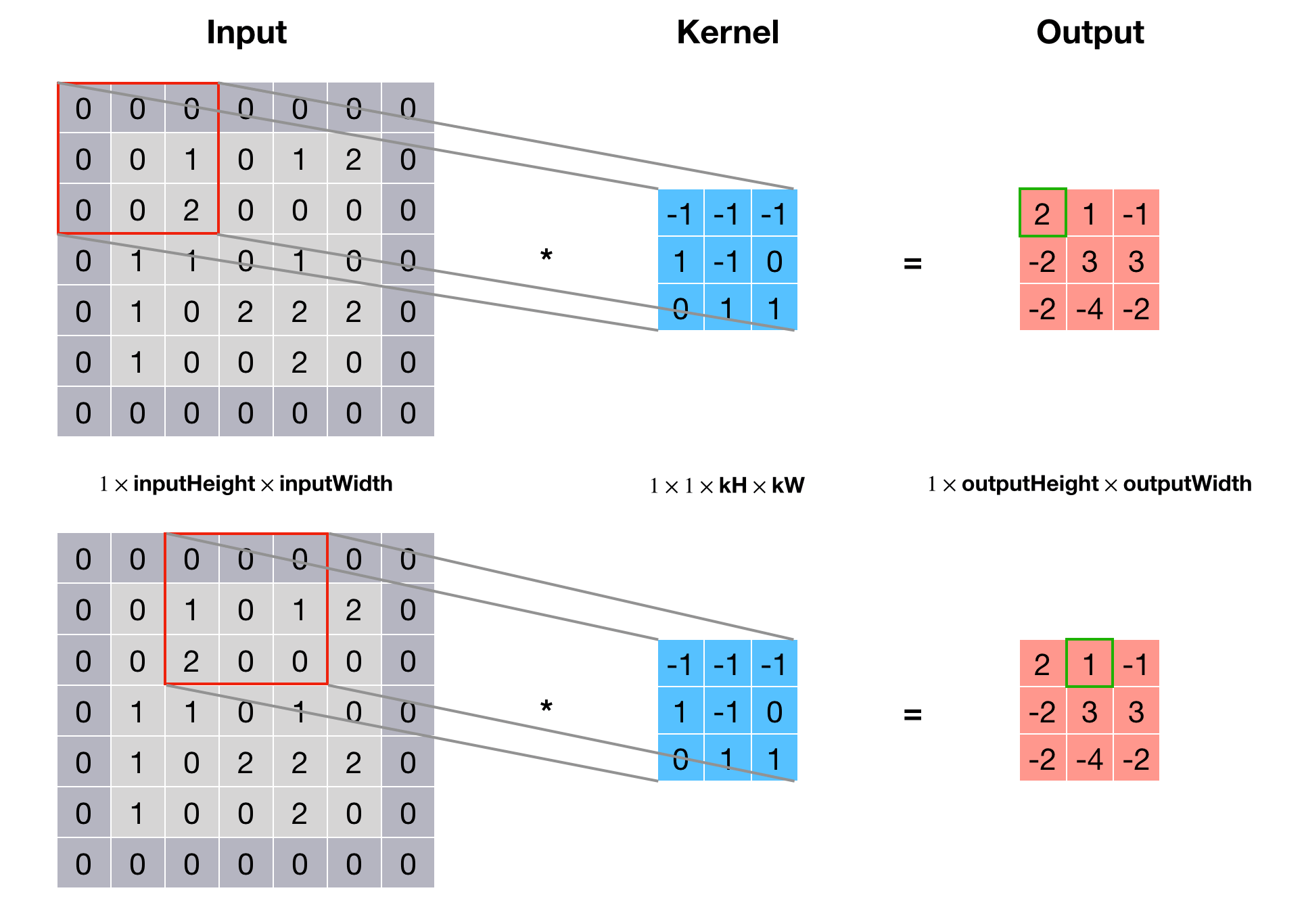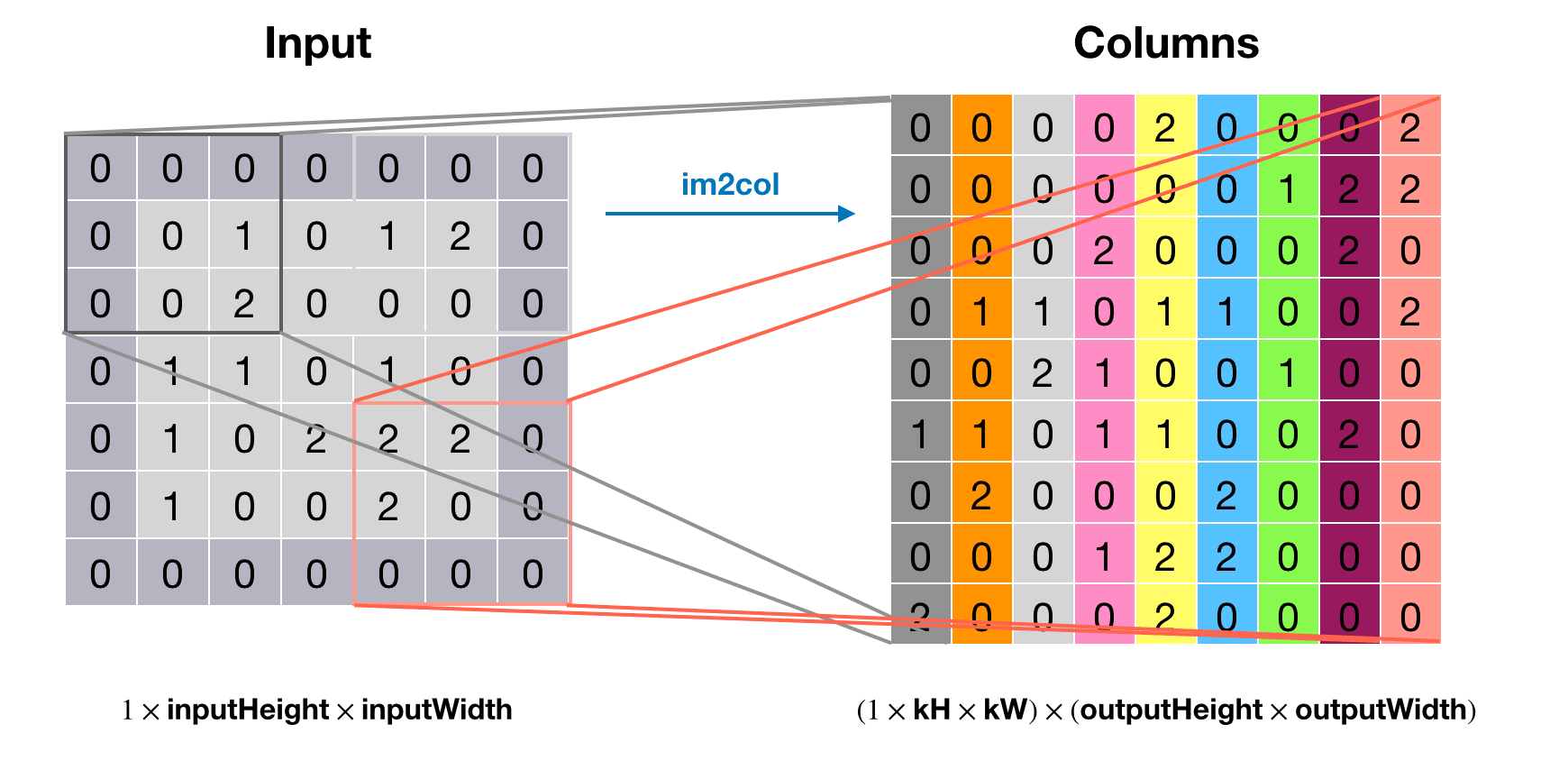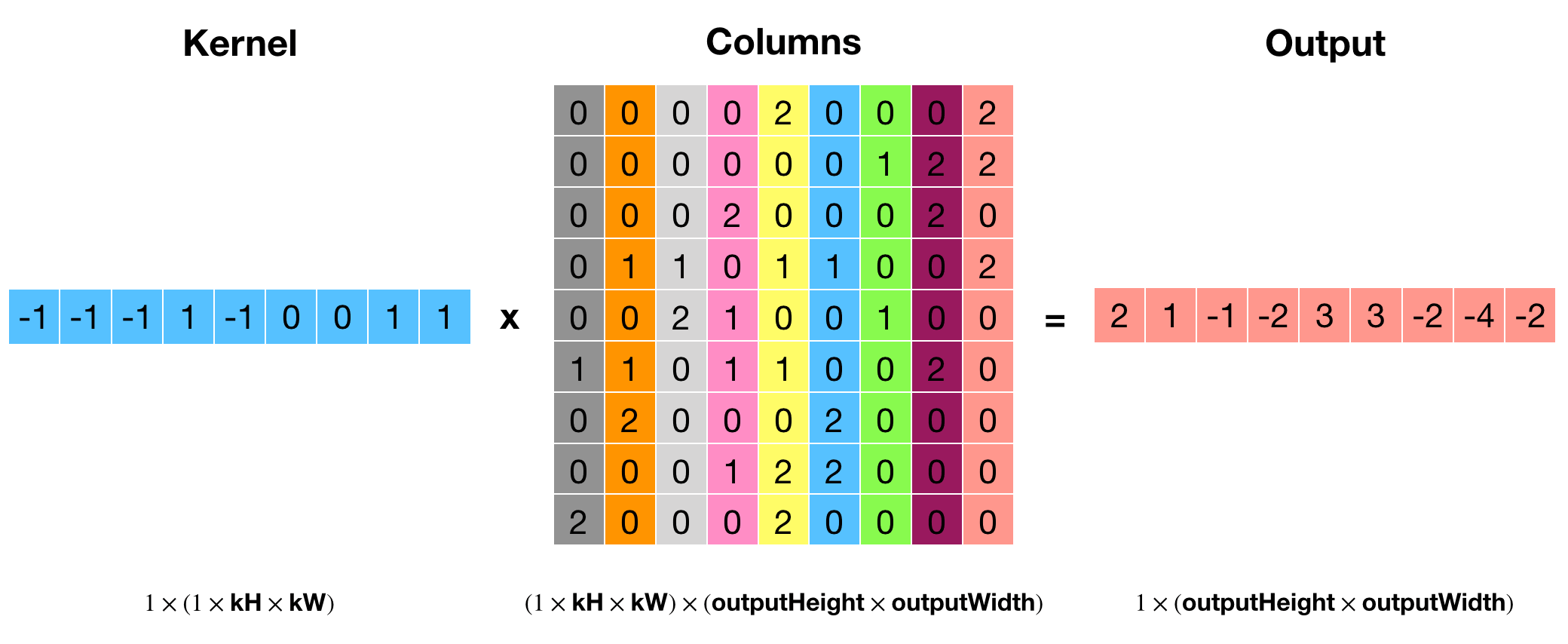#### 反向传播

• gradOutput 是相对于输出的梯度，即 $\delta^l$，大小与前向传播的输出 output 相同；
• gradInput 是相对于输入的梯度，即 $\delta^{l-1}$，是这个函数需要计算的对象，大小与输入 input 相同；
• gradColumns 是个列矩阵，用于存储临时的梯度，大小为 $(\text{nInputPlane}\times\text{kH}\times\text{kW})\times(\text{outputHeight}\times\text{outputWidth})$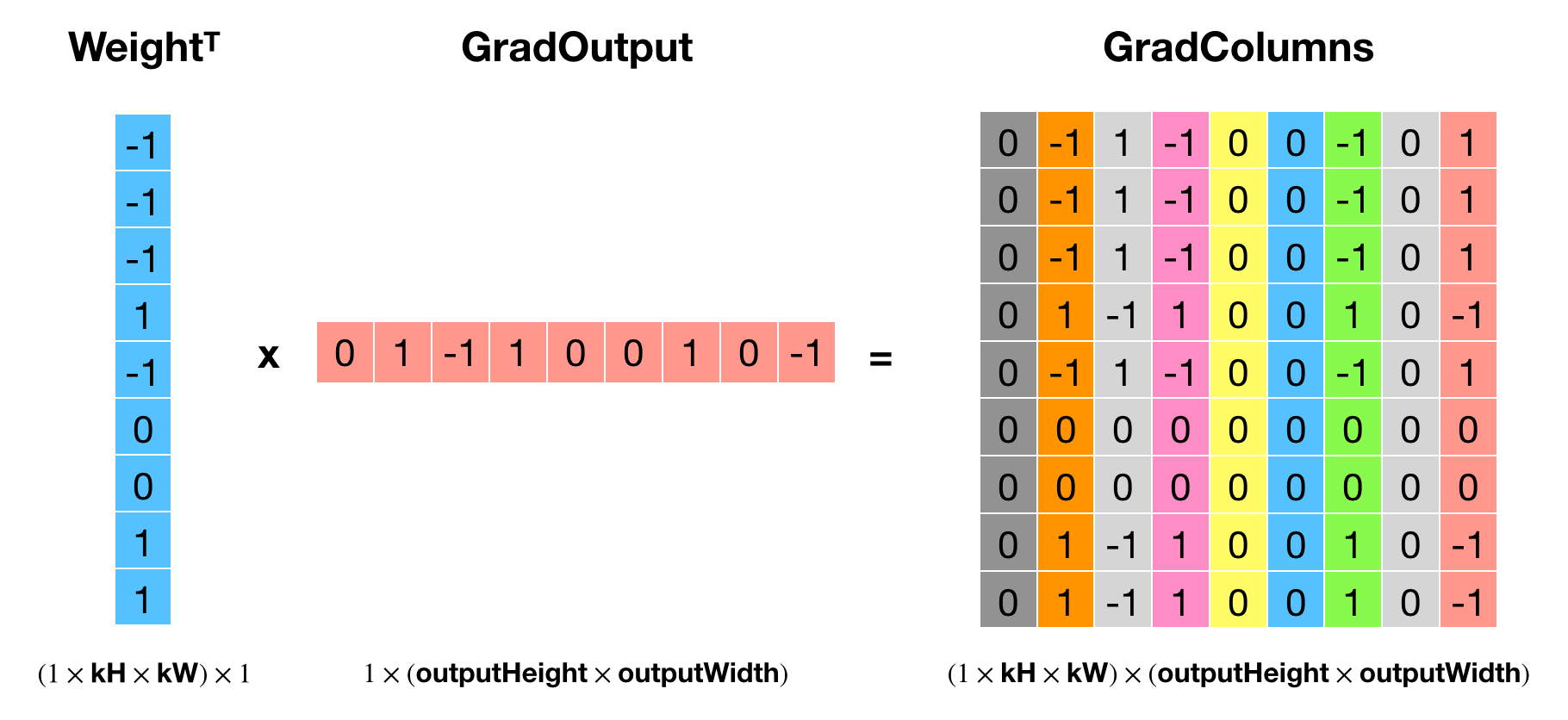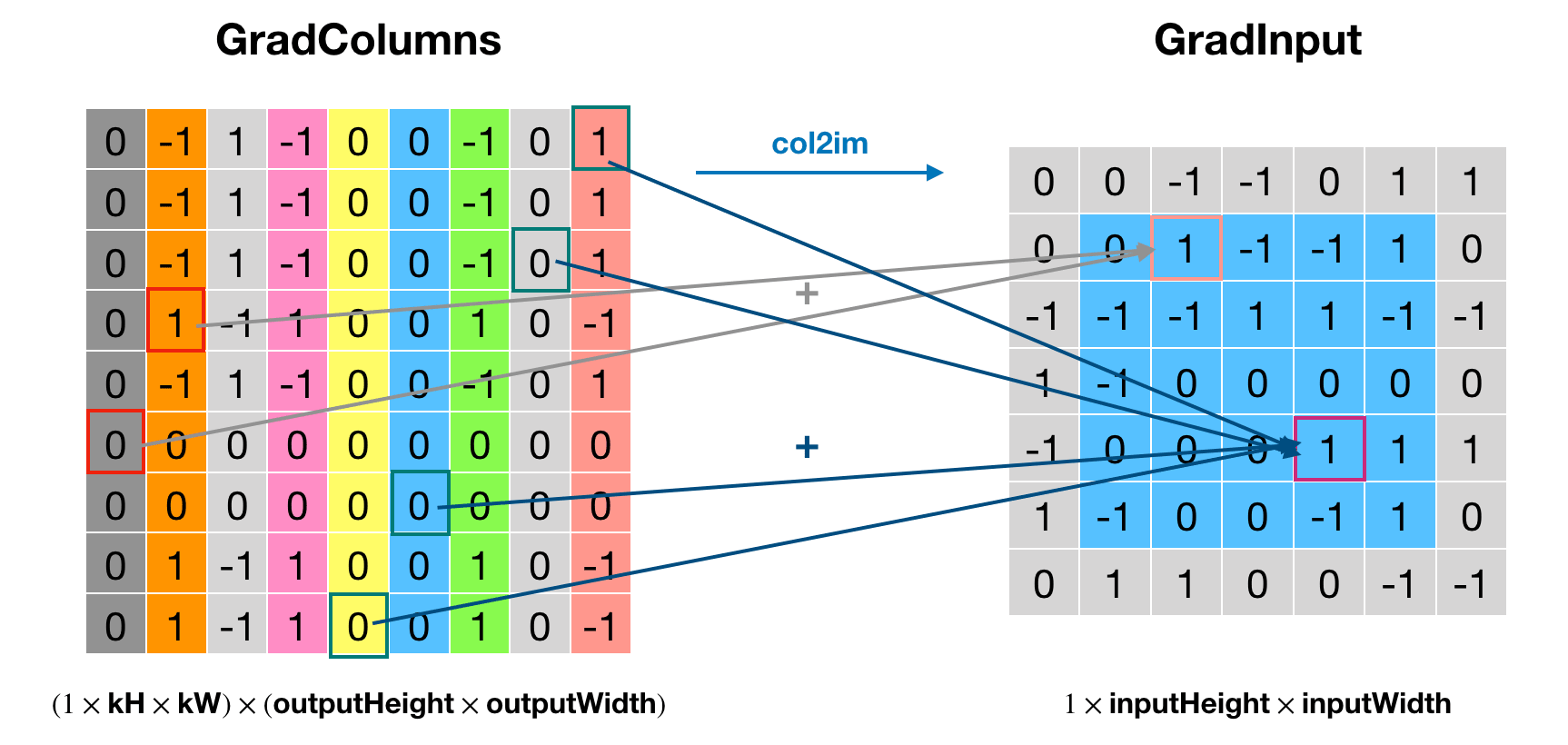• gradWeight 是权重的梯度，是这个函数需要计算的对象，大小和权重相同；
• gradBias 是偏置的梯度，也是函数需要计算的对象，大小与偏置相同（就是一个Vector）
• columns 是用来存储 im2col 的结果，因为要计算卷积，所以要把输入展开
• scale_ 是学习速率（learning rate）

## ATen

• ATen/core/Tensor.h定义了at::Tensor类型，这个是C++前端以及更上层的API都在用的Tensor类型，它的成员内有一个TensorImpl impl_，提供底层实现；
• 实现和封装了有关Tensor的所有操作，并根据数据类型和设备进行自动派发；
• 使用Python脚本生成ATen API。

つづく